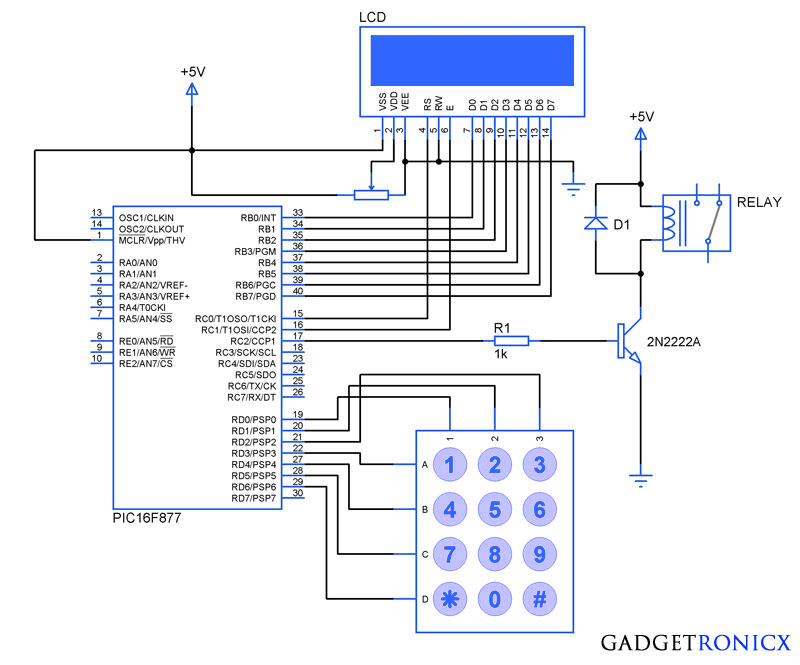# Electronic Code locking system using PIC 16F877 MircocontrollerElectronic Combination lock PIC Mircocontroller

Electronic code locking system is extremely useful in protecting our precious possessions and can be installed anywhere with bit of engineering in it. We are widely familiar with the Password based e-locks and might have installed in our house. But we are going for Electronic locks made by any company when you can make one by your own.

This project demonstrates you how to make a PIC microcontroller based simple digital lock and also explains the programming behind it.

## WHAT YOU NEED:

1. PIC 16F877 Microcontroller
2. 4×3 Keypad – Key Input
3. 16×2 LCD – Status Indicator
4. 5V Relay – Activator

Additionally you need a POT which is used to adjust the contrast of the LCD, A transistor to drive the relay since the current obtained from a Pin of Microcontroller is very less. A diode to prevent the reverse flow of current which might damage the controller.

## DESIGN OF ELECTRONIC CODE LOCKING SYSTEM:

The design of the above Embedded Project is pretty straight forward, you need to interface a LCD to PORT B and Keypad to the PORT D of the Controller. If you are not familiar with it then i suggest you to go through this Interfacing LCD and Keypad with PIC Microcontroller. Now lets see how the system is designed to work as a Locker.

• “*” Key – This key is meant to initialize the system, when the system is turned ON the Controller will scan only this key and pressing this key will enable you to enter the password for your locker.
• “#” Key – When you are done with your locker , you should press this key which will turn off the system and turning off the relay as well.

The remaining keys are meant to feed the character values to the Microcontroller and in turn the Microcontroller will analyze the characters. Based on the Pre defined password in the Controller it will compare the input with it. Thereby it will recognize the correct or incorrect password input.

## CODE:

The below code was built using CCS compiler for the PIC Microcontroller
```#include<16F877.h>
#include<stdio.h>
#bit led=0x05.0
#bit TRIS_led=0x85.0
#byte lcd=0x06
#byte TRIS_lcd=0x86
#bit rs=0x07.0
#bit TRIS_rs=0x87.0
#bit en=0x07.1
#bit TRIS_en=0x87.1
#bit relay=0x07.2
#bit TRIS_relay=0x87.2
#bit C1=0x08.0
#bit C2=0x08.1
#bit C3=0x08.2
#bit R1=0x08.3
#bit R2=0x08.4
#bit R3=0x08.5
#bit R4=0x08.6
#bit TRIS_C1=0x88.0
#bit TRIS_C2=0x88.1
#bit TRIS_C3=0x88.2
#bit TRIS_R1=0x88.3
#bit TRIS_R2=0x88.4
#bit TRIS_R3=0x88.5
#bit TRIS_R4=0x88.6
void display(unsigned char a,int b);  //LCD subroutine
char pswd;
unsigned char welcome_msg="Welcome";
char c;
int flag,i,count,j;
void main()
{
TRIS_lcd=TRIS_en=TRIS_rs=TRIS_led=TRIS_relay=0; //Directions set
TRIS_R1=TRIS_R2=TRIS_R3=TRIS_R4=count=0;
TRIS_C1=TRIS_C2=TRIS_C3=1;
while(TRUE)
{
{
if(c=='*')                                    //Initialize condition
{
flag=1;                                       //Flag set to scan other keys
count=0;
display(0x01,0);
display(0x38,0);
display(0x0f,0);
display(0x80,0);
for(i=0;i<=13;i++)
{
display(open_msg[i],1);
}
display(0xc0,0);
}
else if(c=='#')                         //Turning off condition
{
count=0;
relay=0;
display(0x01,0);
display(0x0c,0);
}
else
{
display('*',1);
pswd[count]=c;                   //Storing input in new arrays
count=count+1;
check();
}
}
}
}
void display(unsigned char a,int b)
{
lcd=a;
rs=b;
en=1;
delay_ms(10);
en=0;
delay_ms(10);
}
{
if(flag==0)                            //Waiting for Initialization
{
while(TRUE)
{
R4=1;
R1=R2=R3=0;
if(C1==1)
{
while(C1==1);
count=0;
return '*';
}
if(C3==1)
{
while(C3==1);
count=0;
return '#';
}
}
}
else if(flag==1)
{
{
R1=1;
R2=R3=R4=0;
if(C1==1)
{
while(C1==1);
return '1';
}
if(C2==1)
{
while(C2==1);
return '2';
}
if(C3==1)
{
while(C3==1);
return '3';
}
R2=1;
R1=R3=R4=0;
if(C1==1)
{
while(C1==1);
return '4';
}
if(C2==1)
{
while(C2==1);
return '5';
}
if(C3==1)
{
while(C3==1);
return '6';
}
R3=1;
R1=R2=R4=0;
if(C1==1)
{
while(C1==1);
return '7';
}
if(C2==1)
{
while(C2==1);
return '8';
}
if(C3==1)
{
while(C3==1);
return '9';
}
R4=1;
R1=R2=R3=0;
if(C1==1)
{
while(C1==1);
return '*';
}
if(C2==1)
{
while(C2==1);
return '0';
}
if(C3==1)
{
while(C3==1);
return '#';
}
}
}
}
void check()
{
if(count>3)                 //Input exceeds count 3 will execute comparison
{
flag=count=0;
j=strcmp(pswd,password);     //Comparison of input and Predefined pswd
if(j==0)
{
relay=1;                         //Turning relay on
display(0x01,0);
display(0x80,0);
for(i=0;i<=6;i++)
{display(welcome_msg[i],1);}
}
else
{
relay=0;
display(0x01,0);
display(0x80,0);
for(i=0;i<=13;i++)
{display(close_msg[i],1);}
}
}
}```

### NOTE:

• It can be done by making the trial value to increase for every wrong password input and checking the condition whether it exceeds the desired trial value.
• Once it exceeds the desired trial value disable the keypad scan and display message “No more trials” in the LCD.
• You can also add speaker to sound a alarm once the trial exceeds to alert the people nearby.

## JLCPCB - Only \$2 for PCB Prototype (Any Color)

Up to \$20 shipping discount on first order now: https://jlcpcb.com/quote

Electronic code Locker
main.hex
Version: 1
3.0 KB
Details12 Followers

Most reacted comment
43 Comment authorsRecent comment authors
Subscribe
Notify ofGuest
hariGuest
yassine bellil

can you give me bluetooth code to send e msg to my cell phone when the ultrasonic detect something !!!Guest
Kwaku

hello frank, at what frequency is the micro controller working at ? 8MHz or 4MHzGuest
rabah

hi Donald
I need help to add a password on my projects;;;; keypad and LCD interfacing a 16F877A PIC;;;;; with Micro C pro for PIC languageGuest
rahul

sir, am getting errors if I run it in MPLABx software, could you tell me any other software in which i can run this.Guest
hamza

Frank I Need your help on a project. contact me hamzainam459@gmail.comGuest
Woon

May i get assembly language of this program please.Guest
hannah

hi..can i know what type of diode did yo used??GuestwpDiscuz